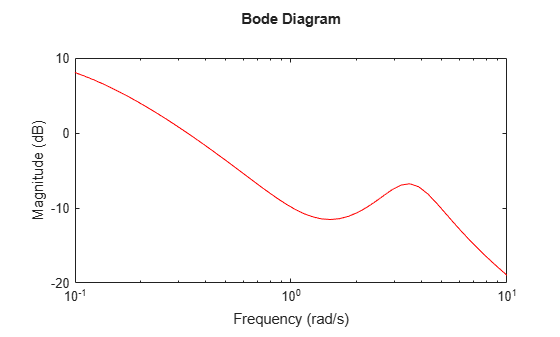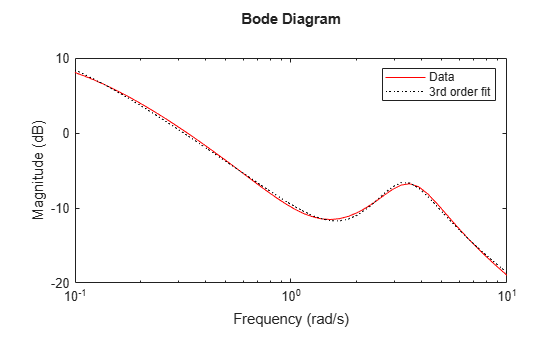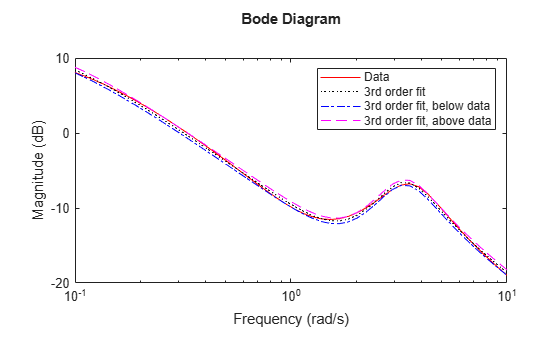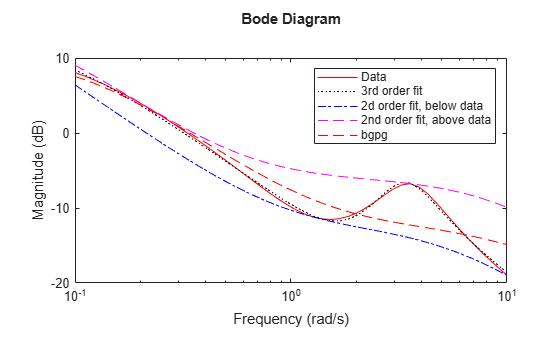fitmagfrd

Fit frequency response magnitude data with minimum-phase state-space model using log-Chebyshev magnitude design

Syntax

B = fitmagfrd(A,N)
B = fitmagfrd(A,N,RD)
B = fitmagfrd(A,N,RD,WT)
B = fitmagfrd(A,N,RD,WT,C)

Description

B = fitmagfrd(A,N) is a stable, minimum-phase ss object, with state-dimension N, whose frequency response magnitude closely matches the magnitude data in A. A is a 1-by-1 frd object, and N is a nonnegative integer.

B = fitmagfrd(A,N,RD) forces the relative degree of B to be RD. RD must be a nonnegative integer whose default value is 0. You can specify the default value for RD by setting RD to an empty matrix.

B = fitmagfrd(A,N,RD,WT) uses the magnitude of WT to weight the optimization fit criteria. WT can be a double, ss or frd. If WT is a scalar, then it is used to weight all entries of the error criteria (A-B). If WT is a vector, it must be the same size as A, and each individual entry of WT acts as a weighting function on the corresponding entry of (A-B). The default value for WT is 1, and you can specify it by setting WT to an empty matrix.

B = fitmagfrd(A,N,RD,WT,C) enforces additional magnitude constraints on B, specified by the values of C.LowerBound and C.UpperBound. These can be empty, double or frd (with C.Frequency equal to A.Frequency). If C.LowerBound is non-empty, then the magnitude of B is constrained to lie above C.LowerBound. No lower bound is enforced at frequencies where C.LowerBound is equal to -inf. Similarly, the UpperBound field can be used to specify an upper bound on the magnitude of B. If C is a double or frd (with C.Frequency equal to A.Frequency), then the upper and lower bound constraints on B are taken directly from A as:

• if C(w) == –1, then enforce abs(B(w)) <= abs(A(w))

• if C(w) == 1, then enforce abs(B(w)) >= abs(A(w))

• if C(w) == 0, then no additional constraint

where w denotes the frequency.

Examples

collapse all

Create frequency response magnitude data from a fifth-order system.

sys = tf([1 2 2],[1 2.5 1.5])*tf(1,[1 0.1]);
sys = sys*tf([1 3.75 3.5],[1 2.5 13]);
omega = logspace(-1,1);
sysg = abs(frd(sys,omega));
bodemag(sysg,'r');Fit the magnitude data with a minimum-phase, stable third-order system.

ord = 3;
b1 = fitmagfrd(sysg,ord);
b1g = frd(b1,omega);
bodemag(sysg,'r',b1g,'k:');
legend('Data','3rd order fit');Fit the magnitude data with a third-order system constrained to lie below and above the given data.

C2.UpperBound = sysg;
C2.LowerBound = [];
b2 = fitmagfrd(sysg,ord,[],[],C2);
b2g = frd(b2,omega);
C3.UpperBound = [];
C3.LowerBound = sysg;
b3 = fitmagfrd(sysg,ord,[],[],C3);
b3g = frd(b3,omega);
bodemag(sysg,'r',b1g,'k:',b2g,'b-.',b3g,'m--')
legend('Data','3rd order fit','3rd order fit, below data',...
'3rd order fit, above data')Fit the magnitude data with a second-order system constrained to lie below and above the given data.

ord = 2;
C2.UpperBound = sysg;
C2.LowerBound = [];
b2 = fitmagfrd(sysg,ord,[],sysg,C2);
b2g = frd(b2,omega);
C3.UpperBound = [];
C3.LowerBound = sysg;
b3 = fitmagfrd(sysg,ord,[],sysg,C3);
b3g = frd(b3,omega);
bgp = fitfrd(genphase(sysg),ord);
bgpg = frd(bgp,omega);
bodemag(sysg,'r',b1g,'k:',b2g,'b-.',b3g,'m--',bgpg,'r--')
legend('Data','3rd order fit','2d order fit, below data',...
'2nd order fit, above data','bgpg')Limitations

This input frd object must be either a scalar 1-by-1 object or, a row, or column vector.

Algorithms

fitmagfrd uses a version of log-Chebyshev magnitude design, solving

min f     subject to (at every frequency point in A):
|d|^2 /(1+ f/WT) < |n|^2/A^2 < |d|^2*(1 + f/WT)

plus additional constraints imposed with C. n, d denote the numerator and denominator, respectively, and B = n/d. n and d have orders (N-RD) and N, respectively. The problem is solved using linear programming for fixed f and bisection to minimize f. An alternate approximate method, which cannot enforce the constraints defined by C, is B = fitfrd(genphase(A),N,RD,WT).

References

Oppenheim, A.V., and R.W. Schaffer, Digital Signal Processing, Prentice Hall, New Jersey, 1975, p. 513.

Boyd, S. and Vandenberghe, L., Convex Optimization, Cambridge University Press, 2004.